Get instant live expert help with Excel or Google Sheets“My Excelchat expert helped me in less than 20 minutes, saving me what would have been 5 hours of work!”

#### Post your problem and you’ll get Expert help in seconds.

Your message must be at least 40 characters
Our professional Expert are available now. Your privacy is guaranteed.

# Count numbers by range with COUNTIFS

While working with Excel, we are able to determine the number of values that fall within a specified range by using the COUNTIFS function. The COUNTIFS function counts the number of cells that meet one or more criteria.  This step by step tutorial will assist all levels of Excel users in counting numbers by range with COUNTIFS.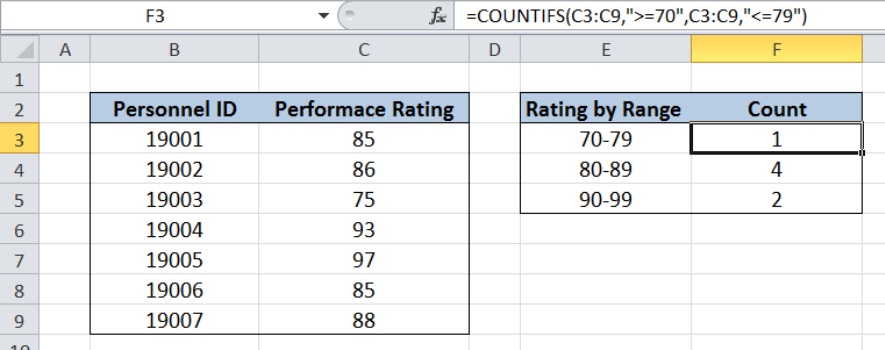Figure 1. Final result: Count cells by range using COUNTIFS

Final formula: `=COUNTIFS(C3:C9,">=70",C3:C9,"<=79")`

## Syntax of COUNTIFS Function

`=COUNTIFS(criteria_range1, criteria1, [criteria_range2, criteria2]…)`

Parameters:

• Criteria_range1: the data range that will be evaluated using the criteria1
• Criteria1:  the criteria or condition that determines which cells will be counted
• Criteria_range2 and criteria2 are optional; only applied when there are more than one criteria as specified

## Setting up Our Data

Our table contains a list of Personnel ID (column B) and Performance Rating (column C).  Cells E3:E5 contains our criteria which classifies the Performance Rating into three ranges: 70-79, 80-89 and 90-99.  The resulting count per range will be reflected in cells F3:F5.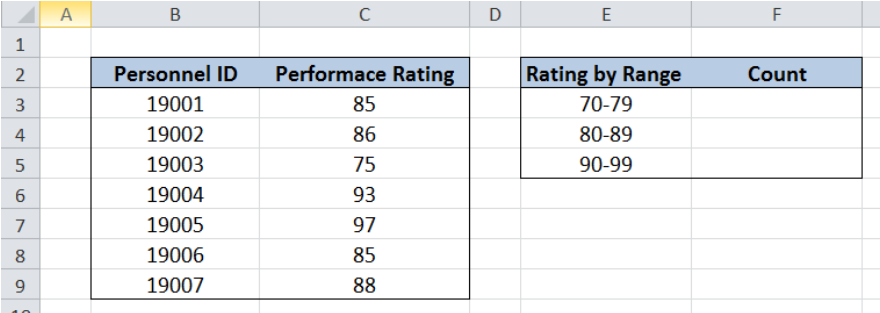Figure 2. Sample data to count numbers by range using COUNTIFS

## Count Numbers by Range using COUNTIFS

We want to count the performance ratings per specified range.

### Count Ratings Between 70 and 79

To count the number of ratings between the range of 70 to 79, we follow these steps:

Step 1.  Select cell F3

Step 2.  Enter the formula: `=``COUNTIFS(C3:C9,">=70",C3:C9,"<=79")`

Step 3: Press ENTER

The range for our data set is C3:C9.  Our formula has two criteria.  It counts the cells in column C  with values between 70 and 79. The symbol “>=” means “greater than or equal to” while “<=” means “less than or equal to”.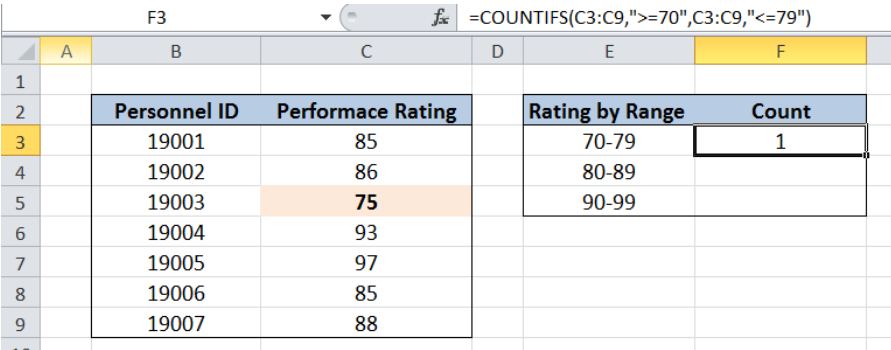Figure 3. Using the COUNTIFS function to count numbers between the range 70 and 79

The formula returns the value 1, which means that there is only one value between 70 and 79 in our data.

### Count Ratings Between 80 and 89

To count the number of ratings between the range of 80 to 89, we follow these steps:

Step 1.  Select cell F4

Step 2.  Enter the formula: `=``COUNTIFS(C3:C9,">=80",C3:C9,"<=89")`

Step 3: Press ENTER

The procedure is the same as in our previous example.  Only this time, the criteria is different because the lowest value is 80, and the highest value is 89.  Our formula counts the cells in column C  with values between 80 and 89.

The formula returns the value 4, which means that there are four values between 80 and 89, as highlighted below.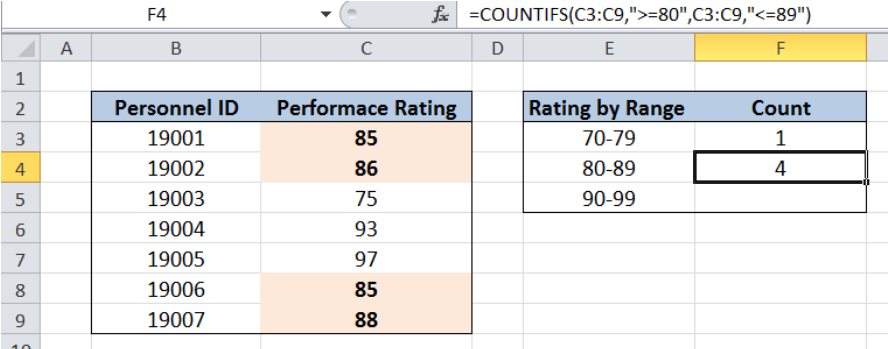Figure 4. Using the COUNTIFS function to count numbers between 80 and 89

### Count Ratings Between 90 and 99

To count the number of ratings between the range of 90 to 99, we follow the same procedure but entering this formula in cell F5:

`=COUNTIFS(C3:C9,">=90",C3:C9,"<=99")`

The only difference is the specified criteria, which is between the range of 90 and 99.  Our formula returns the value 2, for the ratings 93 and 97 as highlighted below.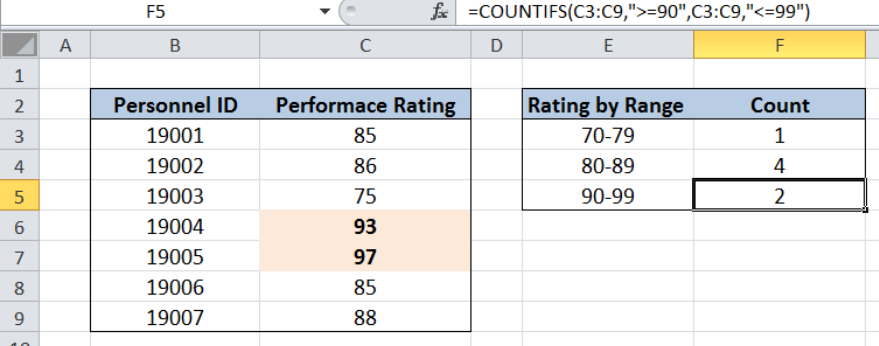Figure 5. Using the COUNTIFS function to count numbers between 90 and 99

Most of the time, the problem you will need to solve will be more complex than a simple application of a formula or function. If you want to save hours of research and frustration, try our live Excelchat service! Our Excel Experts are available 24/7 to answer any Excel question you may have. We guarantee a connection within 30 seconds and a customized solution within 20 minutes.

### Did this post not answer your question? Get a solution from connecting with the expert.Another blog reader asked this question today on Excelchat:
Solution examplesi need a formula to find values in column f in worksheet 1 and count them.
Solved by B. A. in 20 minsI have a spreadsheet that contains a list of names/IDs and I want to generate a formula that inserts into a cell the number of times a name or ID appears on that list. Either insert a column into that spreadsheet or transfer that info into a column on a different spreadsheet. Either way would be fine.
Solved by O. L. in 18 minsi need a formula to count values that meet two conditions: one if a value is contained in a cell and another one is cells aren't empty
Solved by X. Q. in 20 minsGreetings, I am trying to find a formula in excel that will return the number of students attending courses. My data is on one sheet and my report will be on another sheet. The report is formated with the students list in a coulmn (far left), the courses are list in a row (top). My data is formatted with all columns, courses in column in "A" and students in column "B". Thanks
Solved by E. C. in 27 minsin range b2:o2, I am trying to write a formula to count if the values are equal to 1 thru 27.
Solved by E. L. in 52 mins## Subscribe to Excelchat.coAnother blog reader asked this question today on Excelchat: